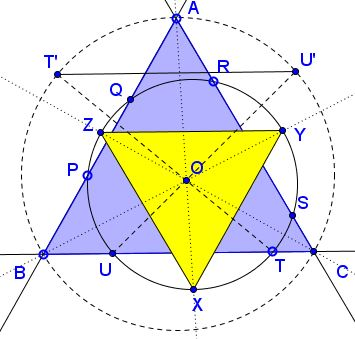# Homothety in Equilateral Triangles

### Problem

A circle meets the sides of an equilateral $\Delta ABC$ in points $P,Q$ (on $AB),$ $R,S$ (on $AC),$ and $T,U$ (on $BC).$ $X,Y,Z$ are the midpoints of arcs $PQ,$ $RS,$ $TU,$ respectively.Prove that $\Delta XYZ$ is equilateral.

### Proof 1

Any two circles are homothetic. Let $O$ be the center of homothety that maps the given circle $\omega=(PQRSTU)$ onto the circumcircle $(ABC).$ Let that homothety map $T$ to $T'$ and $U$ to $U'.$Then $TU\parallel T'U',$ i.e., $BC\parallel T'U'.$ But, $\Delta ABC$ being equilateral, $A$ is the midpoint of the arcs $BC$ and so it is also the midpoint of the arc $T'U'.$ Since homothety preserves angles, the image of $X$ (the midpoint of arc $TU$ of $\omega)$ is the midpoint of arc $T'U'$ of $(ABC)$ which is $A.$ Similarly, $Y$ is mapped on $B$ and $Z$ is mapped on $C.$ The inverse mapping is also a homothety, hence preserves angles, and maps the equilateral $\Delta ABC$ on an equilateral triangle, but the image of $\Delta ABC$ is $\Delta XYZ,$ implying that the latter is also equilateral.

### Proof 2

Let $G$ be the center of $\omega:$ $GX=GY=GZ$ and $GX\perp BC,$ $GY\perp AC,$ $GZ\perp AB.$It follows that $\angle XGZ=\angle YGZ=\angle XGY.$ In particular $\angle OXY=\angle OXZ=30^{\circ},$ so that $\angle YXZ=60^{\circ}.$ Similarly, $\angle XYZ=\angle XZY=60^{\circ}.$

### Acknowledgment

The above problem has been posted by Miguel Ochoa Sanchez at the CutTeKnotMath facebook page; the second solution is by Emil Stoyanov; Leo Giugiuc supplied a trigonometric solution.

• Angle Trisectors on Circumcircle
• Equilateral Triangles On Sides of a Parallelogram
• Pompeiu's Theorem
• Pairs of Areas in Equilateral Triangle
• The Eutrigon Theorem
• Equilateral Triangle in Equilateral Triangle
• Seven Problems in Equilateral Triangle
• Spiral Similarity Leads to Equilateral Triangle
• Parallelogram and Four Equilateral Triangles
• A Pedal Property in Equilateral Triangle
• Miguel Ochoa's van Schooten Like Theorem
• Two Conditions for a Triangle to Be Equilateral
• Incircle in Equilateral Triangle
• When Is Triangle Equilateral: Marian Dinca's Criterion
• Barycenter of Cevian Triangle
• Excircle in Equilateral Triangle
• Converse Construction in Pompeiu's Theorem
• Wonderful Trigonometry In Equilateral Triangle
• 60o Angle And Importance of Being The Other End of a Diameter
• One More Property of Equilateral Triangles
• Van Khea's Quickie
• Equilateral Triangle from Three Centroids
•